# Number of Unique Paths

Number of Unique Paths: This is a standard dynamic problem which can be featured in any interview coding.
Submitted by Radib Kar, on February 16, 2020

Description:

In this article, we are going to see a standard dynamic problem which can be featured in any interview coding.

Problem statement:

Given a M X N matrix with initial position at top-left cell, find the number of possible unique paths to reach the bottom right cell of the matrix from the initial position. Possible moves can be either down or right at any point in time.

```    Input:
The first line contains an integer T,
depicting total number of test cases.
Then following T lines contains two integers
m and n depicting the size of the grid.

Output:
Print the number of unique paths to reach
bottom-right cell from the top-left cell.
```

Example with explanation:

```    Input:
2
3 3
3 4

Output:
6
10
```

So, for the first test case, M=3, n=3

Grid is like,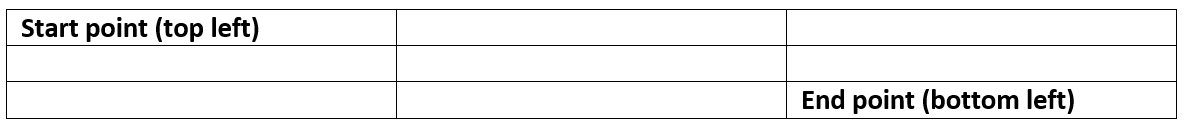1. (0,0)->(0,1)->(0,2)->(1,2)->(2,2)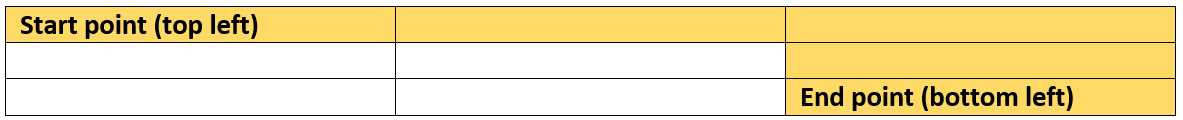2. (0,0)->(0,1)->(1,1)->(1,2)->(2,2)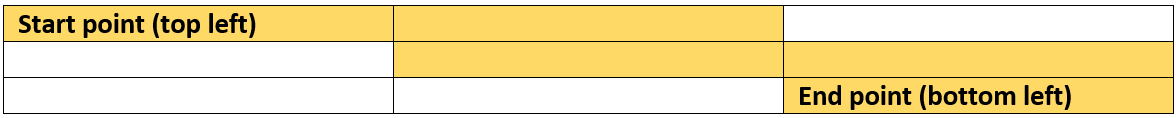3. (0,0)->(0,1)->(1,1)->(2,1)->(2,2)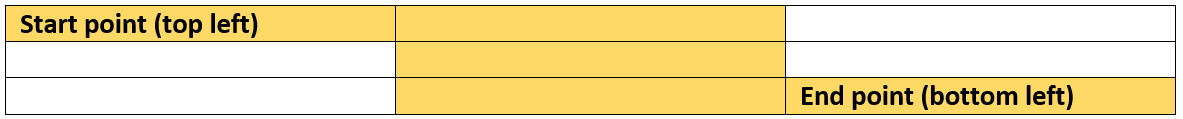4. (0,0)->(1,0)->(2,0)->(2,1)->(2,2)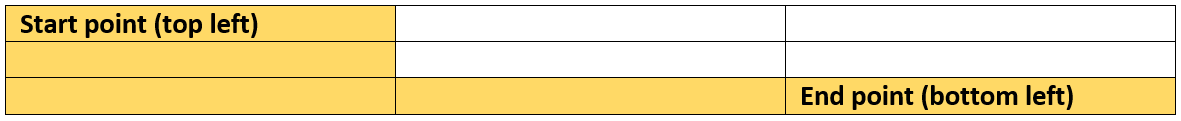5. (0,0)->(1,0)->(1,1)->(1,2)->(2,2)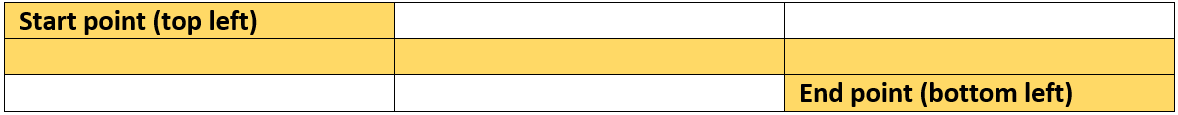6. (0,0)->(1,0)->(1,1)->(2,1)->(2,2)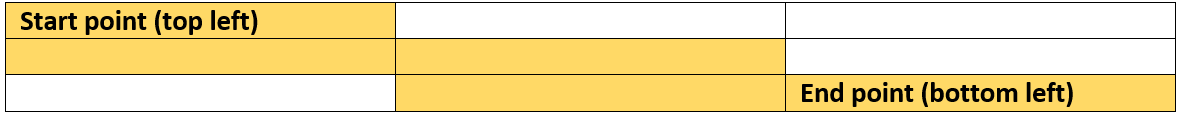This are the possible unique paths and hence count is 6.

Given a matrix with an initial position at the top-left cell, find the number of possible unique paths to reach the bottom-right cell of the matrix from the initial position. Possible moves can be either or at any point in time.

Recursion Algorithm:

```    Of course, we can generate a recursion algorithm.
Since the only possible moves are down and right
from any point, we can write a recursive relation,

f(m,n) = f(m-1,n) + f(m,n-1)
Where f(m,n) = number of unique path for grid size m,n
f(m-1,n) = number of unique path for grid size [(m-1),n]
a down move from the end point of this will result in f(m,n)
f(m,n-1) = number of unique path for grid size [m,(n-1)]
a right move from the end point of this will result in f(m,n)
Hence, f(m,n) is summation of f(m-1,n) and f(m,n-1)
```

So, the algorithm can be:

```    Function Uniquepaths(m,n):
1)  If m==0 & n==0 return 0
2)  If m == 0
Then return 1
3)  If n==0
Then return 1
4) Return Uniquepaths(m-1,n)+Uniquepaths(m,n-1)
```

But this would generate many overlapping subproblems, which will be computed again and again increasing the time complexity. Hence, we can convert the recursion to dynamic Programming.

Dynamic Programming approach:

```    1)  Create a 2D array DP with size m, n
2)  Initialize DP =0 which is similar to step 1 in our previous function.
3)  for i=1 to n-1
DP[i]=1
end for
This is similar to step2 in our previous function
4)  for i=1 to m-1
DP[i]=1
end for
This is similar to step3 in our previous function

5)  Compute the whole DP table
for i=1 to m-1
for j=1 to n-1
DP[i][j]=DP[i-1][j]+DP[i][j-1]
end for
end for
This is similar to step 4 in our previous recursive function
6)  Return DP[m-1][n-1] which is the result.
```

C++ Implementation:

```#include <bits/stdc++.h>
using namespace std;

int uniqueRoute(int m,int n){
int DP[m][n];

memset(DP,0,sizeof(DP));

//base cases
DP=0;

for(int i=1;i<m;i++)
DP[i]=1;

for(int i=1;i<n;i++)
DP[i]=1;

//compute dp table
for(int i=1;i<m;i++){
for(int j=1;j<n;j++){
DP[i][j]=DP[i-1][j]+DP[i][j-1];
}
}
return DP[m-1][n-1];
}

int main()
{
int t,n,m;

cout<<"Enter number of testcases\n";
cin>>t;

for(int i=0;i<t;i++){
cout<<"Enter grid size, m & n\n";
cin>>m>>n;
cout<<"Number of unique paths from topleft to bottom right: "<<uniqueRoute(m,n)<<endl;
}

return 0;
}
```

Output

```Enter number of testcases
2
Enter grid size, m & n
3 3
Number of unique paths from topleft to bottom right: 6
Enter grid size, m & n
3 4
Number of unique paths from topleft to bottom right: 10
```

What's New (MCQs)

Top Interview Coding Problems/Challenges!

Languages: » C » C++ » C++ STL » Java » Data Structure » C#.Net » Android » Kotlin » SQL
Web Technologies: » PHP » Python » JavaScript » CSS » Ajax » Node.js » Web programming/HTML
Solved programs: » C » C++ » DS » Java » C#
Aptitude que. & ans.: » C » C++ » Java » DBMS
Interview que. & ans.: » C » Embedded C » Java » SEO » HR
CS Subjects: » CS Basics » O.S. » Networks » DBMS » Embedded Systems » Cloud Computing
» Machine learning » CS Organizations » Linux » DOS
More: » Articles » Puzzles » News/Updates

© https://www.includehelp.com some rights reserved.